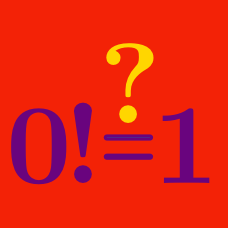Number Theory

# Number Theory Common Misconceptions: Level 3 ChallengesCan an even number, divided by another even number, times another even number ever equal an odd number?

If "yes," then find three numbers that work.
If "no," then why not?

Note that the three even numbers can be different numbers.

For integral choices of $x$ and $y$, $\text{LCM}(x, y) \leq xy.$

Is the above statement true or false?

Clarification: The $\text{LCM}$ is the Lowest Common Multiple of two numbers.

$N$ is a positive integer such that $10N$ leaves a remainder of 70 when divided by 100; that is, $10N \equiv 70 \bmod{100}.$ What is the remainder when $N$ is divided by 100?

True or False: Every odd number greater than 1 is the smallest member of a primitive Pythagorean triple.

Note: A primitive Pythagorean triple is a triple of positive integers $(a,b,c)$ such that $a^2+b^2 = c^2$ and $\gcd(a,b,c) = 1.$

$\large\text{Irrational}^{\text{Irrational}}= \text{Irrational}$

Is the above equation always true for irrational numbers? Each irrational number can be different.

×